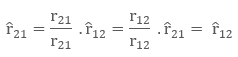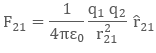We already know that two like charges repel each other while two, unlike charges, attract each other. thus, it is evident that a force acts between two charges. This force is called 'electric force'. The electric force between like charges is repulsive and that between two, unlike charges, attract. If electric charges are situated in a vacuum, even then electric force acts between them.

In 1785, Coulomb, on the basis of experiments, gave a law regarding the force acting between two charges known as Coulomb's Law.

## Coulomb's Law Definition

According to this law, two stationary point - charges repel or attract each other with a force which is directly proportional to the product of the charges and inversely proportional to the square of the distance between them. This force is along the line joining the two charges.

## Coulomb's Law Equation

EquationSymbol breakdownMeaning in words
open vertical bar, F, start subscript, E, end subscript, close vertical bar, equals, k, open vertical bar, start fraction, q, start subscript, 1, end subscript, q, start subscript, 2, end subscript, divided by, r, start superscript, 2, end superscript, end fraction, close vertical barF, start subscript, E, end subscript is an electric force, k is the Coulomb’s law constant, q, start subscript, 1, end subscript and q, start subscript, 2, end subscript are the charges, and r is the distance between the charges.The magnitude of the electric force between q, start subscript, 1, end subscript and q, start subscript, 2, end subscript is directly proportional to the magnitude of the charges and inversely proportional to the square of the distance between them. This equation is known as Coulomb’s Law.

## Coulomb's Law Explanation

If we took two charges q1  and q2 are situated at a distance r apart then the force acting between them is given by

FQ1Q2r2,

F=kQ1Q2r2,

where k is proportionality constant which depends on the units used for charges, distance, and force. If the unit of force is newton (N), unit of distance is meter (m), unit of charge is coulomb (C) and both of the charges are situated in a vacuum then the experimental value of k comes to be

9,0×109 N⋅m2⋅C2

## Coulomb's Law in vector form

The physical quantities are of mainly two types scalar (with the only magnitude) and vectors (those quantities with magnitude and direction). Force is a vector quantity and having both magnitude and direction. Coulomb’s law can be re-written in the form of vectors.

Let there will be two charges q1 and q2, with position vectors r1 and r2 respectively. Now, since both the charges are of same nature, there is a repulsive force between them. Let the force on the q1 charge due to q2 be F12 and force on the q2 charge due to q1charge be F21. The vector from q1 to q2 is r21 vector.

r21 = r2 – r1

To denote the direction of a vector from position vector r1 to  r2 , and from r2 to r1 as:Now, the force on both the charge q2 due to q1, in vector form is:The above formula is showing the vector form of Coulomb’s Law.

## Some Important Points Regarding Coulomb's Law

1) Coulomb's law is based on experimental results.
2) The law holds only for point charges.
3) Both the charges exert equal and opposite forces on each other.
4) The force between two charges is not changed by the presence of other charges.
5) The law is only applicable for charges at rest.

## Limitations of Coulomb's Law

1) It only depends upon experimental results.
2) The law holds only for point charges.
3) We can use the formula if the charges are static ( in rest position)

## Fundamental units

Q1Q

1.2.3.